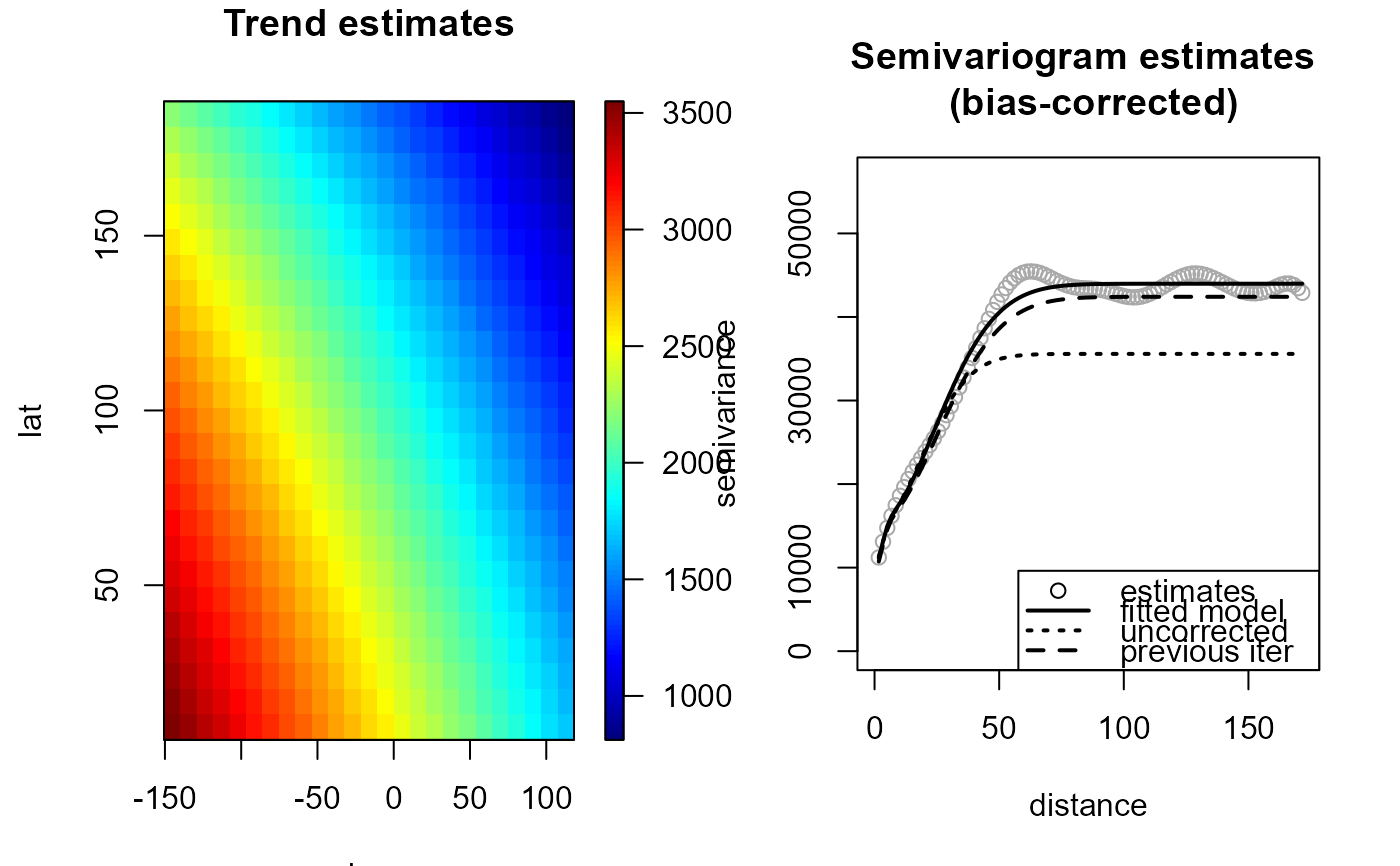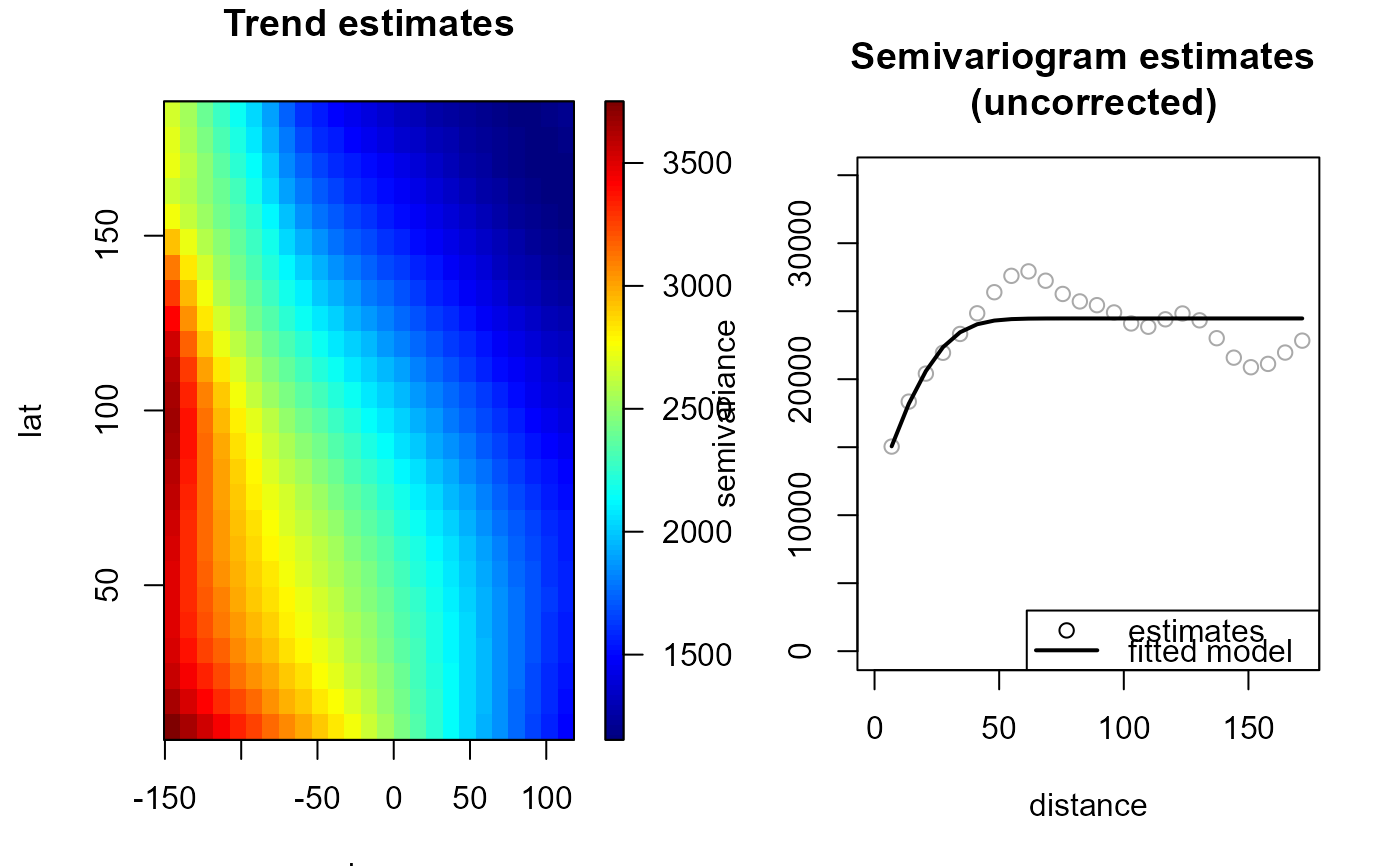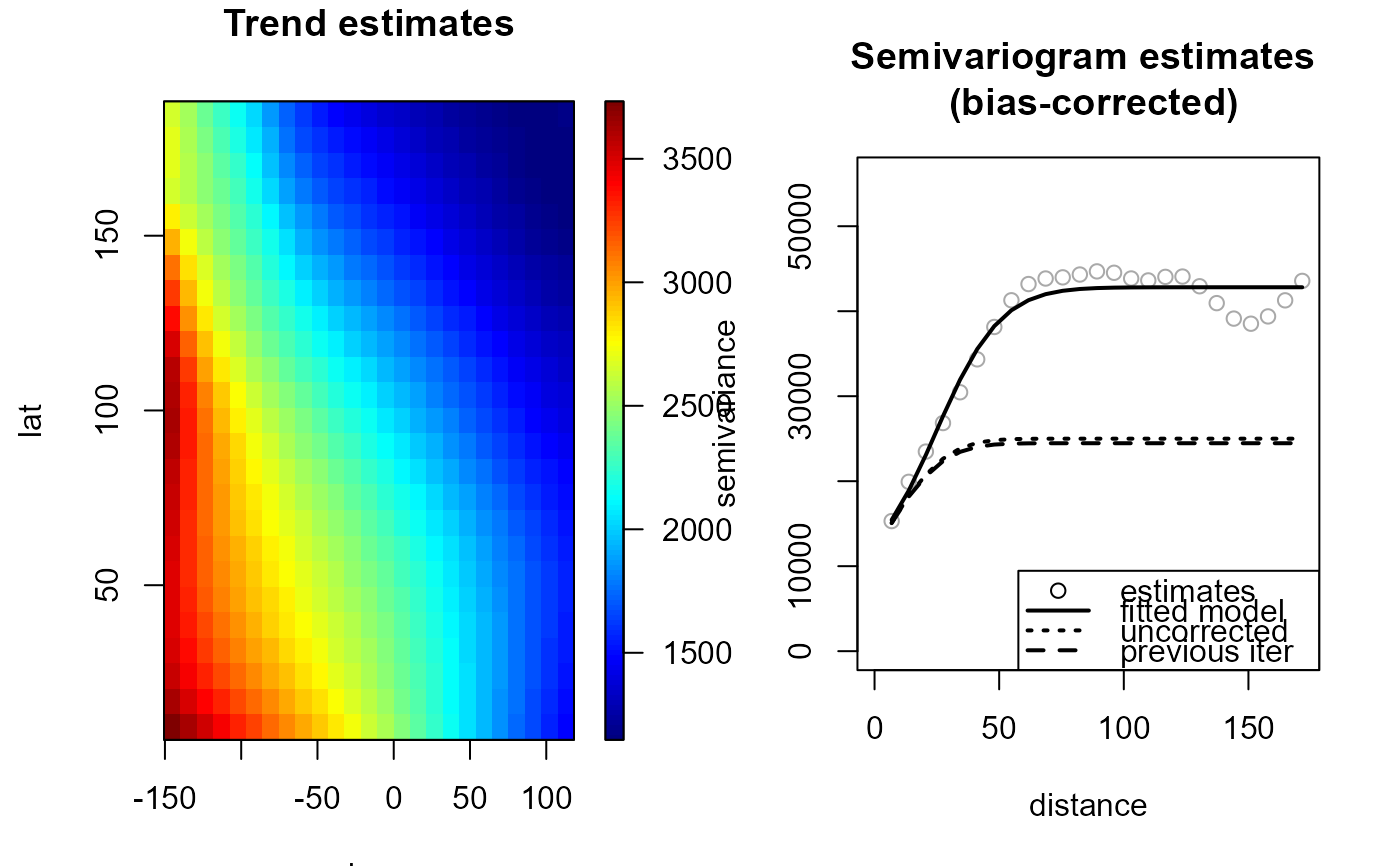Fits a nonparametric (isotropic) geostatistical model (jointly estimates the trend and the variogram) by calling locpol, np.svariso.corr (or np.svariso ) and fitsvar.sb.iso iteratively. At each iteration, the trend estimation bandwith is updated by a call to h.cv.

np.fitgeo(x, ...)

# S3 method for default
np.fitgeo(
x,
y,
nbin = NULL,
iter = 2,
h = NULL,
tol = 0.05,
set.NA = FALSE,
h.svar = NULL,
corr.svar = iter > 0,
maxlag = NULL,
nlags = NULL,
dk = 0,
svm.resid = FALSE,
hat.bin = corr.svar,
warn = FALSE,
plot = FALSE,
window = NULL,
...
)

# S3 method for locpol.bin
np.fitgeo(
x,
svm,
iter = 1,
tol = 0.05,
h.svar = svm$esv$locpol$h, dk = 0, corr.svar = TRUE, svm.resid = FALSE, hat.bin = corr.svar, warn = FALSE, plot = FALSE, ... ) # S3 method for fitgeo np.fitgeo( x, iter = 1, tol = 0.05, h.svar = x$svm$esv$locpol$h, dk = x$svm$par$dk,
corr.svar = TRUE,
svm.resid = FALSE,
hat.bin = corr.svar,
warn = FALSE,
plot = FALSE,
...
)

## Arguments

x a (data) object used to select a method. further arguments passed to h.cv (trend bandwith selection parameters). vector of data (response variable). vector with the number of bins on each dimension. maximum number of interations (of the whole algorithm). initial bandwidth matrix for trend estimation (final bandwith if iter = 1). relative convergence tolerance (semivariogram). logical. If TRUE, sets the bin averages corresponding to cells without data to NA. bandwidth matrix for variogram estimation. logical; if TRUE (default), a bias-corrected semivariogram estimate is computed (see np.svariso.corr). If FALSE the (uncorrected) residual variogram is computed (the traditional approach in geostatistics). maximum lag. Defaults to 55% of largest lag. number of lags. Defaults to 101. dimension of the Shapiro-Botha variogram model (see fitsvar.sb.iso). logical; if TRUE, the fitted (uncorrected) residual semivariogram model is computed and returned (this parameter has no effect when corr.svar = FALSE). logical; if TRUE, the hat matrix of the binned data is returned. logical; sets the handling of warning messages in bandwith selection (h.cv). logical; if TRUE, semivariogram estimates obtained at each iteration are plotted. spatial window (values outside this window will be masked), currently an sp-object of class extending SpatialPolygons. (fitted) variogram model (object of class fitsvar or svarmod).

## Value

Returns an object of class fitgeo (extends np.geo). A locpol.bin object with the additional (some optional) 3 components:

svm

fitted variogram model (object of class fitsvar).

svm0

(if requested) fitted residual variogram model (object of class fitsvar).

residuals

model residuals.

## Details

Currently, only isotropic semivariogram estimation is supported.

If parameter h is not specified, h.cv is called with the default values (modified CV) to set it. If parameter h.svar is not specified, is set to 1.5*h.cv.svar.bin()$h. Setting corr.svar = TRUE may be very slow (and memory demanding) when the number of data is large (note also that the bias in the residual variogram decreases when the sample size increases). ## See also locpol, fitsvar.sb.iso, np.svar, np.svariso.corr, np.geo. ## Examples  geomod <- np.fitgeo(aquifer[,1:2], aquifer$head, svm.resid = TRUE)
plot(geomod)# Uncorrected variogram estimator
geomod0 <- np.fitgeo(aquifer[,1:2], aquifer\$head, iter = 1, corr.svar = FALSE)
plot(geomod0)# Additional iteration with bias-corrected variogram estimator
geomod1 <- np.fitgeo(geomod0, corr.svar = TRUE, svm.resid = TRUE)
plot(geomod1)